>

#### NCERT Solutions for Class 11th: Ch 3 Motion In A Straight Line Physics Science

3.15. In Exercises 3.13 and 3.14, we have carefully distinguished between average speed and magnitude of average velocity. No such distinction is necessary when we consider instantaneous speed and magnitude of velocity. The instantaneous speed is always equal to the magnitude of instantaneous velocity. Why?

Instantaneous velocity is given by the first derivative of distance with respect to time i.e. ,
vIn = dx / dt
Here, the time interval dt is so small that it is assumed that the particle does not change its direction of motion. As a result, both the total path length and magnitude of displacement become equal is this interval of time.
Therefore, instantaneous speed is always equal to instantaneous velocity.

3.16. Look at the graphs (a) to (d) (Fig. 3.20) carefully and state, with reasons, which of these cannot possibly represent one-dimensional motion of a particle.(a) The given x-t graph, shown in (a), does not represent one-dimensional motion of the particle. This is because a particle cannot have two positions at the same instant of time.

(b) The given v-t graph, shown in (b), does not represent one-dimensional motion of the particle. This is because a particle can never have two values of velocity at the same instant of time.

(c) The given v-t graph, shown in (c), does not represent one-dimensional motion of the particle. This is because speed being a scalar quantity cannot be negative.

(d) The given v-t graph, shown in (d), does not represent one-dimensional motion of the particle. This is because the total path length travelled by the particle cannot decrease with time.

Page No: 58

3.17. Figure 3.21 shows the x-t plot of one-dimensional motion of a particle. Is it correct to say from the graph that the particle moves in a straight line for t < 0 and on a parabolic path for t > 0? If not, suggest a suitable physical context for this graph.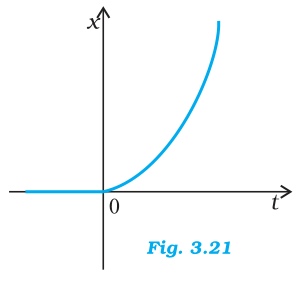No, because the x-t graph does not represent the trajectory of the path followed by a particles. From the graph, it is noted that at t=0, x=0.

3.18. A police van moving on a highway with a speed of 30 km h-1 fires a bullet at a thief’s car speeding away
in the same direction with a speed of 192 km h-1. If the muzzle speed of the bullet is 150 m s-1, with what speed does the bullet hit the thief’s car ? (Note: Obtain that speed which is relevant for damaging the thief’s car).

Speed of the police van, vp = 30 km/h = 8.33 m/s
Muzzle speed of the bullet, vb = 150 m/s
Speed of the thief’s car, vt = 192 km/h = 53.33 m/s
Since the bullet is fired from a moving van, its resultant speed can be obtained as:
= 150 + 8.33 = 158.33 m/s
Since both the vehicles are moving in the same direction, the velocity with which the bullet hits the thief’s car can be obtained as:
vbt = vb – vt
= 158.33 – 53.33 = 105 m/s

3.19. Suggest a suitable physical situation for each of the following graphs (Fig 3.22):(a) The given x-t graph shows that initially a body was at rest. Then, its velocity increases with time and attains an instantaneous constant value. The velocity then reduces to zero with an increase in time. Then, its velocity increases with time in the opposite direction and acquires a constant value. A similar physical situation arises when a football (initially kept at rest) is kicked and gets rebound from a rigid wall so that its speed gets reduced. Then, it passes from the player who has kicked it and ultimately gets stopped after sometime.

(b) In the given v-t graph, the sign of velocity changes and its magnitude decreases with a passage of time. A similar situation arises when a ball is dropped on the hard floor from a height. It strikes the floor with some velocity and upon rebound, its velocity decreases by a factor. This continues till the velocity of the ball eventually becomes zero.

(c) The given a-t graph reveals that initially the body is moving with a certain uniform velocity. Its acceleration increases for a short interval of time, which again drops to zero. This indicates that the body again starts moving with the same constant velocity. A similar physical situation arises when a hammer moving with a uniform velocity strikes a nail.

3.20. Figure 3.23 gives the x-t plot of a particle executing one-dimensional simple harmonic motion. (You will learn about this motion in more detail in Chapter14). Give the signs of position, velocity and acceleration variables of the particle at t = 0.3 s, 1.2 s, - 1.2 s.Negative, Negative, Positive (at t = 0.3 s)
Positive, Positive, Negative (at t = 1.2 s)
Negative, Positive, Positive (at t = –1.2 s)
For simple harmonic motion (SHM) of a particle, acceleration (a) is given by the relation:
a = – ω2x ω → angular frequency … (i)
t = 0.3 s
In this time interval, x is negative. Thus, the slope of the x-t plot will also be negative. Therefore, both position and velocity are negative. However, using equation (i), acceleration of the particle will be positive.
t = 1.2 s
In this time interval, x is positive. Thus, the slope of the x-t plot will also be positive. Therefore, both position and velocity are positive. However, using equation (i), acceleration of the particle comes to be negative.
t = – 1.2 s
In this time interval, x is negative. Thus, the slope of the x-t plot will also be negative. Since both x and t are negative, the velocity comes to be positive. From equation (i), it can be inferred that the acceleration of the particle will be positive.

3.21. Figure 3.24 gives the x-t plot of a particle in one-dimensional motion. Three different equal intervals of time are shown. In which interval is the average speed greatest, and in which is it the least? Give the sign of average velocity for each interval.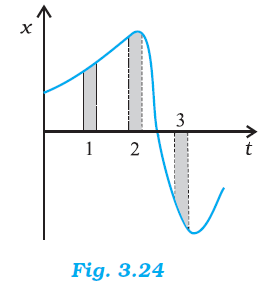Interval 3 (Greatest), Interval 2 (Least)
Positive (Intervals 1 & 2), Negative (Interval 3)
The average speed of a particle shown in the x-t graph is obtained from the slope of the graph in a particular interval of time.
It is clear from the graph that the slope is maximum and minimum restively in intervals 3 and 2 respectively. Therefore, the average speed of the particle is the greatest in interval 3 and is the least in interval 2. The sign of average velocity is positive in both intervals 1 and 2 as the slope is positive in these intervals. However, it is negative in interval 3 because the slope is negative in this interval.

Page No: 59

3.22. Figure 3.25 gives a speed-time graph of a particle in motion along a constant direction. Three equal intervals of time are shown. In which interval is the average acceleration greatest in magnitude ? In which interval is the average speed greatest ? Choosing the positive direction as the constant direction of motion, give the signs of v and a in the three intervals. What are the accelerations at the points A, B, C and D?

Average acceleration is greatest in interval 2
Average speed is greatest in interval 3
v is positive in intervals 1, 2, and 3
a is positive in intervals 1 and 3 and negative in interval 2
a = 0 at A, B, C, D
Acceleration is given by the slope of the speed-time graph. In the given case, it is given by the slope of the speed-time graph within the given interval of time.
Since the slope of the given speed-time graph is maximum in interval 2, average acceleration will be the greatest in this interval.
Height of the curve from the time-axis gives the average speed of the particle. It is clear that the height is the greatest in interval 3. Hence, average speed of the particle is the greatest in interval 3.

In interval 1:
The slope of the speed-time graph is positive. Hence, acceleration is positive. Similarly, the speed of the particle is positive in this interval.

In interval 2:
The slope of the speed-time graph is negative. Hence, acceleration is negative in this interval. However, speed is positive because it is a scalar quantity.

In interval 3:
The slope of the speed-time graph is zero. Hence, acceleration is zero in this interval. However, here the particle acquires some uniform speed. It is positive in this interval.

Points A, B, C, and D are all parallel to the time-axis. Hence, the slope is zero at these points. Therefore, at points A, B, C, and D, acceleration of the particle is zero.

3.23. A three-wheeler starts from rest, accelerates uniformly with 1 m s-2 on a straight road for 10 s, and then moves with uniform velocity. Plot the distance covered by the vehicle during the nth second (n = 1,2,3….) versus n. What do you expect this plot to be during accelerated motion : a straight line or a parabola ?

Straight line
Distance covered by a body in nth second is given by the relation
Dn = u + a (2n - 1)/2     ....(i)
Where,
u = Initial velocity
a = Acceleration
= Time = 1, 2, 3, ..... ,n
In the given case,
u = 0 and a = 1 m/s2
∴ Dn = (2n - 1) / 2     ..... (ii)
This relation shows that:
Dn ∝ … (iii)

Now, substituting different values of n in equation (iii), we get the following table:

 n 1 2 3 4 5 6 7 8 9 10 Dn 0.5 1.5 2.5 3.5 4.5 5.5 6.5 7.5 8.5 9.5

The plot between and Dn will be a straight line shown in below figure: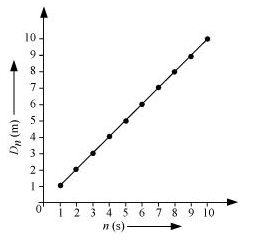3.24. A boy standing on a stationary lift (open from above) throws a ball upwards with the maximum initial speed he can, equal to 49 m s-1. How much time does the ball take to return to his hands? If the lift starts moving up with a uniform speed of 5 m s-1 and the boy again throws the ball up with the maximum speed he can, how long does the ball take to return to his hands ?

Initial velocity of the ball, u = 49 m/s
Acceleration, a = – g = – 9.8 m/s2

Case I:
When the lift was stationary, the boy throws the ball.
Taking upward motion of the ball,
Final velocity, v of the ball becomes zero at the highest point.
From first equation of motion, time of ascent (t) is given as:
v = u +at
t = (v - u/ a
= -49 / -9.8 = 5 s
But, the time of ascent is equal to the time of descent.
Hence, the total time taken by the ball to return to the boy’s hand = 5 + 5 = 10 s.

Case II:
The lift was moving up with a uniform velocity of 5 m/s. In this case, the relative velocity of the ball with respect to the boy remains the same i.e., 49 m/s. Therefore, in this case also, the ball will return back to the boy’s hand after 10 s.

3.25. On a long horizontally moving belt (Fig. 3.26), a child runs to and fro with a speed 9 km h-1 (with respect to the belt) between his father and mother located 50 m apart on the moving belt. The belt moves with a speed of 4 km h-1. For an observer on a stationary platform outside, what is the
(a) speed of the child running in the direction of motion of the belt ?.
(b) speed of the child running opposite to the direction of motion of the belt ?
(c) time taken by the child in (a) and (b) ?
Which of the answers alter if motion is viewed by one of the parents ?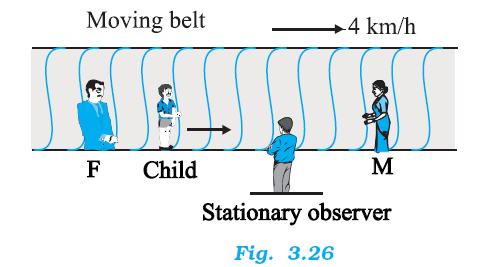(a) Speed of the belt, vB = 4 km/h
Speed of the boy, vb = 9 km/h
Since the boy is running in the same direction of the motion of the belt, his speed (as observed by the stationary observer) can be obtained as:
vbB = vb + vB = 9 + 4 = 13 km/h

(b) Since the boy is running in the direction opposite to the direction of the motion of the belt, his speed (as observed by the stationary observer) can be obtained as:
vbB = vb + (– vB) = 9 – 4 = 5 km/h

(c) Distance between the child’s parents = 50 m
As both parents are standing on the moving belt, the speed of the child in either direction as observed by the parents will remain the same i.e., 9 km/h = 2.5 m/s.
Hence, the time taken by the child to move towards one of his parents is 50 / 2.5 = 20 s

(d) If the motion is viewed by any one of the parents, answers obtained in (a) and (b) get altered. This is because the child and his parents are standing on the same belt and hence, are equally affected by the motion of the belt. Therefore, for both parents (irrespective of the direction of motion) the speed of the child remains the same i.e., 9 km/h.
For this reason, it can be concluded that the time taken by the child to reach any one of his parents remains unaltered.

3.26. Two stones are thrown up simultaneously from the edge of a cliff 200 m high with initial speeds of 15 m s-1 and 30 m s-1. Verify that the graph shown in Fig. 3.27 correctly represents the time variation of the relative position of the second stone with respect to the first. Neglect air resistance and assume that the stones do not rebound after hitting the ground. Take g = 10 m s-2. Give the equations for the linear and curved parts of the plot.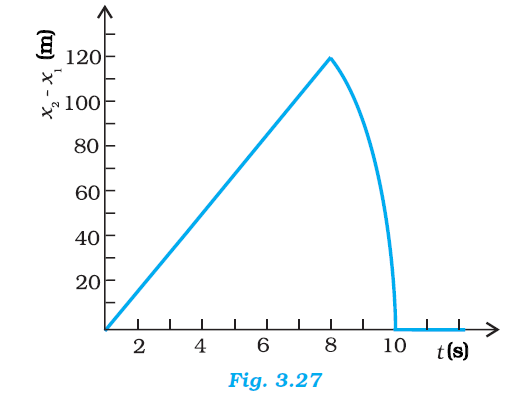For first stone:
Initial velocity, uI = 15 m/s
Acceleration, a = –g = – 10 m/s2

Using the relation,
x1 = x0 + u1t + (1/2)at2
Where, height of the cliff, x0 = 200 m
x1 = 200 + 15t - 5t2      ......(i)
When this stone hits the ground, x1 = 0
∴– 5t+ 15t + 200 = 0
t2 – 3t – 40 = 0
t2 – 8t + 5t – 40 = 0
(t – 8) + 5 (t – 8) = 0
t = 8 s or = – 5 s
Since the stone was projected at time t = 0, the negative sign before time is meaningless.
t = 8 s
For second stone:
Initial velocity, uII = 30 m/s
Acceleration, a = – g = – 10 m/s2

Using the relation,
x2 = x0 + uIIt + (1/2)at2
= 200 + 30t - 5t2........(ii)
At the moment when this stone hits the ground; x2 = 0
– 5t2 + 30 t + 200 = 0
t2 – 6t – 40 = 0
t2 – 10t + 4t + 40 = 0
t (t – 10) + 4 (t – 10) = 0
t (t – 10) (t + 4) = 0
t = 10 s or t = – 4 s
Here again, the negative sign is meaningless.
∴ t = 10 s
Subtracting equations (i) and (ii), we get
x2 - x1 = (200 + 30t -5t2) - (200 + 15t -5t2)
x2 - x1 =15t     ....... (iii)
Equation (iii) represents the linear path of both stones. Due to this linear relation between (x– x1) and t, the path remains a straight line till 8 s.
Maximum separation between the two stones is at t = 8 s.
(x2 – x1)max = 15× 8 = 120 m
This is in accordance with the given graph.
After 8 s, only second stone is in motion whose variation with time is given by the quadratic equation:
x2 – x= 200 + 30t – 5t2
Hence, the equation of linear and curved path is given by
x– x1 = 15t (Linear path)
x2 ­– x1 = 200 + 30t – 5t2 (Curved path)

Page No: 60

3.27. The speed-time graph of a particle moving along a fixed direction is shown in Fig. 3.28. Obtain the distance traversed by the particle between (a) t = 0 s to 10 s, (b) t = 2 s to 6 s.What is the average speed of the particle over the intervals in (a) and (b) ?(a) Distance travelled by the particle = Area under the given graph
= (1/2) × (10 - 0) × (12 - 0) = 60 m
Average speed = Distance / Time = 60 / 10 = 6 m/s

(b) Let s1 and s2 be the distances covered by the particle between time
= 2 s to 5 s and t = 5 s to 6 s respectively.
Total distance (s) covered by the particle in time t = 2 s to 6 s
s = s1 + s2 … (i)

For distance s1:
Let u′ be the velocity of the particle after 2 s and a′ be the acceleration of the particle in t = 0 to t = 5 s.
Since the particle undergoes uniform acceleration in the interval t = 0 to t = 5 s, from first equation of motion, acceleration can be obtained as:
at
Where,
v = Final velocity of the particle
12 = 0 + a′ × 5
a′ = 12 / 5 = 2.4 ms-2
Again, from first equation of motion, we have
at
= 0 + 2.4 × 2 = 4.8 m/s
Distance travelled by the particle between time 2 s and 5 s i.e., in 3 s
s1 = ut + (1/2)at2
= 4.8 × 3 + (1/2) × 2.4 × (3)2
= 25.2 m      ........(ii)

For distance s2:
Let a″ be the acceleration of the particle between time t = 5 s and t = 10 s.
From first equation of motion,
at (where v = 0 as the particle finally comes to rest)
0 = 12 + a″ × 5
a″ = -12 / 5 = - 2.4 ms-2
Distance travelled by the particle in 1s (i.e., between = 5 s and t = 6 s)
s2 = u" t + (1/2)a″ t2
= 12 × 1 + (1/2) (-2.4) × (1)2
= 12 - 1.2 = 10.8 m    .........(iii)

From equations (i), (ii), and (iii), we get
s = 25.2 + 10.8 = 36 m
∴ Average speed = 36 / 4 = 9 m/s

3.28. The velocity-time graph of a particle in one-dimensional motion is shown in Fig. 3.29 :
Which of the following formulae are correct for describing the motion of the particle over the time-interval t1 to t2 :
(a) x(t2 ) = x(t1) + v (t1) (t2 – t1) +(½) a (t2 – t1)2
(b) v(t2 ) = v(t1) + a (t2 – t1)
(c) vaverage = (x(t2) – x(t1))/(t2 – t1)
(d) aaverage = (v(t2) – v(t1))/(t2 – t1)
(e) x(t2 ) = x(t1) + vaverage (t2 – t1) + (½) aaverage (t2 – t1)2
(f) x(t2 ) – x(t1) = area under the v-t curve bounded by the t-axis and the dotted line shown.